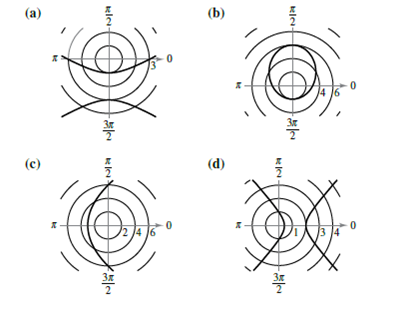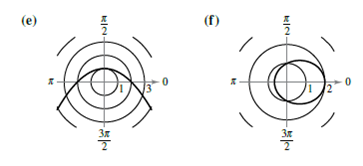Chapter 10.6, Problem 10E

Chapter
Section
Textbook Problem

# Matching In Exercises 7-12, match the polar equation with its correct graph. [The graphs are labeled (a), (b), (c), (d>, (e), and f).]r = 2 1 + sin θ

To determine

The correct match of the given polar equation with its correct graph from the options given.

Explanation

Given: Given options for the graphs are labelled as (a), (b), (c), (d), (e) and (f) as shown below.

Calculation: The given polar equation is r=21+sinθ

Here e=1, so the graph of the given equation is a parabola.

The form of the equation concludes that the directrix is horizontal and it is above the pole

### Still sussing out bartleby?

Check out a sample textbook solution.

See a sample solution

#### The Solution to Your Study Problems

Bartleby provides explanations to thousands of textbook problems written by our experts, many with advanced degrees!

Get Started

#### Expand each expression in Exercises 122. (y3+2y2+y)(y2+2y1)

Finite Mathematics and Applied Calculus (MindTap Course List)

#### In Exercises 49-62, find the indicated limit, if it exists. 62. limx24x22x2+x3

Applied Calculus for the Managerial, Life, and Social Sciences: A Brief Approach

#### In problems 37-48, compute and simplify so that only positive exponents remain. 46.

Mathematical Applications for the Management, Life, and Social Sciences

#### Differentiate the function. G(y)=ln(2y+1)5y2+1

Single Variable Calculus: Early Transcendentals

#### True or False: is a convergent series.

Study Guide for Stewart's Single Variable Calculus: Early Transcendentals, 8th

#### Explain the distinction between a hypothesis and a prediction.

Research Methods for the Behavioral Sciences (MindTap Course List)